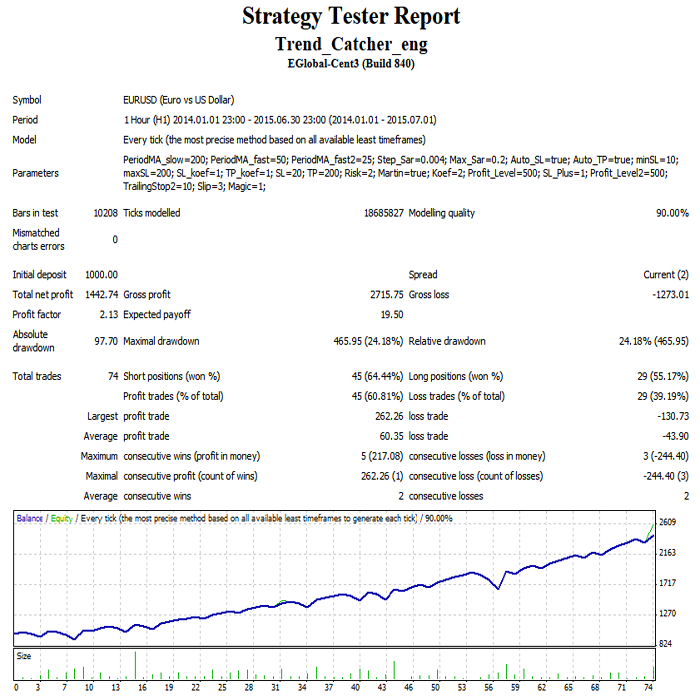Interesting script?
So post a link to it -
let others appraise it

You liked the script? Try it in the MetaTrader 5 terminal# Trend_Catcher - expert for MetaTrader 4

Views:
38438
Rating:
Published:
2015.10.07 10:45
Updated:
2016.11.22 07:32

The EA looks for trend using three MAs (period 200, 50, 25 or other) and opens orders using the Parabolic SAR indicator. There is martingale in an Expert Advisor, the lot is calculated in percents from a deposit depending on Stop Loss.

The updated version has some new adds, user can: 1) choose days for trade, 2) open orders on the inverted signal, 3) disable closing an order on an opposite signal.

Entering the market

• Buy: if current price is higher than point of Parabolic SAR indicator on a current candle, Close price of the previous candle is lower than the previous point of Parabolic SAR indicator, МА is higher than the first slow МА, current price is higher than the second МА with other period.

• Sell: if current price is below than point of Parabolic SAR indicator on a current candle, Close price of the previous candle is higher than the previous point of Parabolic SAR indicator, МА is lower than the first slow МА, current price is lower than the second МА with other period.

• The Expert Advisor closes the order at the opposite signal.

Stop Loss and Take Profit

• If Auto_SL = true, then the Stop Loss is calculated automatically and is set on the point of Parabolic SAR indicator. You can apply the SL_koef coefficient which is multiplied by the distance in points between the level of opening the order and the point of the Parabolic SAR indicator. Example: if the distance from the order price to the point of Parabolic SAR indicator is 20 points and SL_koef = 2, then an order will be opened with a Stop Loss of 40 points, if SL_koef = 0.5, the order will be placed with a Stop Loss of 10 points.

• If Auto_SL = false, Stop Loss will be fixed at the parameter SL in points.

• If Auto_TP = true, Take Profit is calculated automatically by the Stop Loss and multiplied by TP_koef. Example: If TP_koef = 2, it means that the Take Profit will be twice of Stop Loss.

• If Auto_TP = false, the Take Profit is fixed at TP in points.

Lot and martingale settings

• Order lot size is calculated by the percentage of the deposit, based on the Risk setting and Stop Loss. Example: the account balance is \$1,000, Stop Loss is 100 points at Risk = 1 (1 percent of the deposit) we can lose 10 currency units, the lot will be 0.01, at Risk = 10 the lot will be 0.1.

• If Martin = true and the latest order is closed with a loss, the risk of the next trade will be increased by Koef times.

• If Martin = false, then the EA will calculate the lot at the percentage of the current account balance.

Breakeven and Trailing Stop

If the position profit reaches Profit_Level pips, then SL_Plus pips of the profit will be put a Stop Loss.

If the position profit reaches Profit_Level2 pips, at TrailingStop2 distance Stop Loss will be dragged by the price.Translated from Russian by MetaQuotes Software Corp.
Original code: https://www.mql5.com/ru/code/13661Instant Execution New

Instant execution button to close profit orders, close all orders, auto close by trailing parameter. Last update: 21 April 2016. Prev: 8 Dec 2015Squeeze RA V1

My version of Squeeze Indicator for MetaTrader 4. This indicator is based on a strategy mentioned in John Carter's book, Mastering the Trade. It is also a fully improvised version of Squeeze_Break indicator by Des O'Regan.AutoSLTP

This EA will help you to set Stop Loss and Take Profit.Traditional MACD with Momentum indicador and alarms

Traditional MACD indicator with a Momentum indicator and alarms. Allows customization of most of the parameters without having to dive into code.